Mobile QR Code1. (Microwave Embedded Circuit & System (MECAS) Lab. School of Electrical Engineering, Chung-Ang University, Korea)

Injection-locked quadrature voltage-controlled oscillator (IL-QVCO), fourth-order resonator, in-phase and quadrature (I/Q), phase difference, CMOS

## I. INTRODUCTION

In the wireless communication field, an in-phase and quadrature (I/Q) signal is used for modulation methods such as quadrature phase shift keying and quadrature amplitude modulation (1,2). In addition, it can be used to eliminate ghost targets in radar applications (3,4). The frequency band required by wireless communication and radar application is increasing to the millimeter-wave band, and the bandwidth is also expanding. Therefore, a local oscillator must be used to output the millimeter-wave I/Q signal with a wide frequency range.

Fig. 1 shows four I/Q generator topologies: (a) frequency divider, (b) passive phase shifter, (c) quadrature voltage-controlled oscillator (QVCO), and (d) injection-locked (IL)-QVCO. The frequency divider receives the signal of f0 and outputs an I/Q signal, which decreases the frequency (5,6). Thus, it is not suitable for millimeter-wave frequency applications because a frequency that is twice as high is necessary to generate the I/Q signal. In addition, an active phase shifter, such as a polyphase filter (PPF), consists of passive components, including resistors and capacitors, that consume no power (7). The PPF is typically cascaded with different time constant to combat variations in the process, voltage and temperature (PVT). In addition, calibration circuits and driving amplifiers are used to calibrate the phase error and loss (8). QVCOs can output a I/Q signal without an input signal; however, they have a considerably narrow operation frequency range (9-11). The operation frequency ranges of a Colpitts QVCO (9) and a QVCO with tail current injection (10) are 0.217 GHz (4%) and 0.44 GHz (8%), respectively. The self-injection-coupled (SIC)-QVCO (11) and phase-tunable injection-coupled (PTIC)-QVCO (12) also have operation frequency ranges of 0.76 GHz (14%) and 0.6 GHz (6%), respectively.

Fig. 1. I/Q signal generator (a) frequency divider, (b) passive phase shifter, (c) QVCO, (d) IL-QVCO.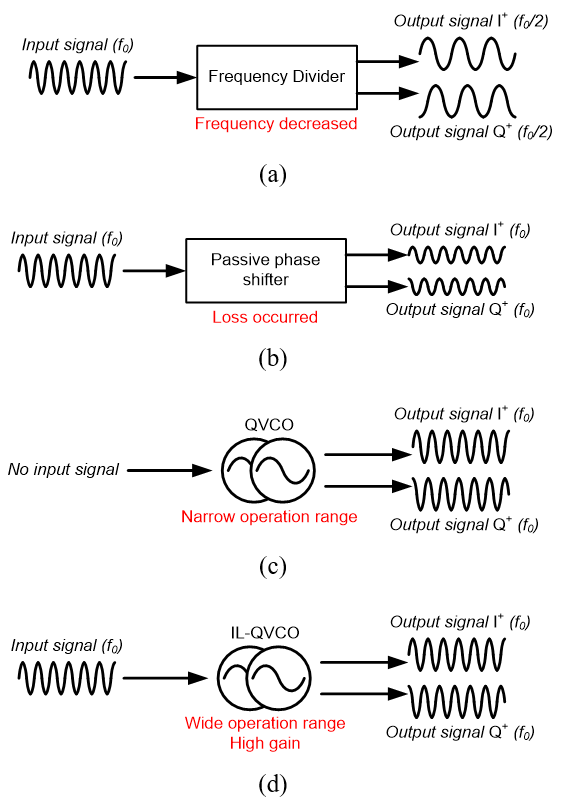Table 1. Design parameters

 Design parameter Value M1, M2, M9, M10 (W/L) 14/0.06 (mm/mm) M3, M4, M5, M6, M11, M12 (W/L) 8/0.06 (mm/mm) M7, M8, M13, M14 (W/L) 60/0.06 (mm/mm) L1, L1 737.5 pH L2, L6 791.3 pH L3, L7 1.5 nH L4, L8 1.44 nH k12, k56 0.29, 0.84 C1, C3 331 fF C2, C4 209 fF

In this study, an injection-locked (IL)-QVCO is proposed that can operate in a wide frequency range. The input signal should be applied because it is injection-locked to the fundamental signal; however, there are no frequency reduction and loss. Furthermore, there is no additional control circuit, such as a capacitor bank. This article is structured as follows: In Section II, the circuit in this work is described. In Sections III and IV, the measurement results and conclusion are presented.

## II. CIRCUIT DESCRIPTION

Fig. 2 shows a schematic of the proposed IL-QVCO that consists of an NMOS cross-coupled pair (M1–4, M9–12), direct injector (M5, 6), fourth-order resonator (L1, 2, L5, 6, C1–4), and output buffer (M7, 8, M13, 14, L3, 4, L7, 8). The differential input signal (Vin+, Vin) is applied to the gate of the injector (M5, 6) through DC blocking capacitors (Cdc). The DC voltage (Vin,DC) is biased through the high-impedance resistor (Rdc). The two nodes of the primary coil (L1, L3) are connected to the feedback injector (M3, 4, M9, 10); they oscillate with a phase difference of 90°. Additionally, in the figure, k represents the coupling coefficient between the primary and secondary coils. The value of k determines the self-resonance point. The I/Q signals (VoutI+, VoutI–, VoutQ+, and VoutQ–) are output from the secondary coil (L2, L6) nodes through the output buffer and have the same frequency as the input signals. In other words, this IL-QVCO is injection-locked to the fundamental signal. The topology of the output buffer is a differential common-source amplifier. The load impedance of the output buffer is matched at 8 GHz. Vbias is generated by an internal self-bias circuit, which is not shown in the figure to reduce complexity. The design parameters are organized in Table 1.

Fig. 3. Load impedance magnitude of the (a) second-order resonator, (b) fourth-order resonator.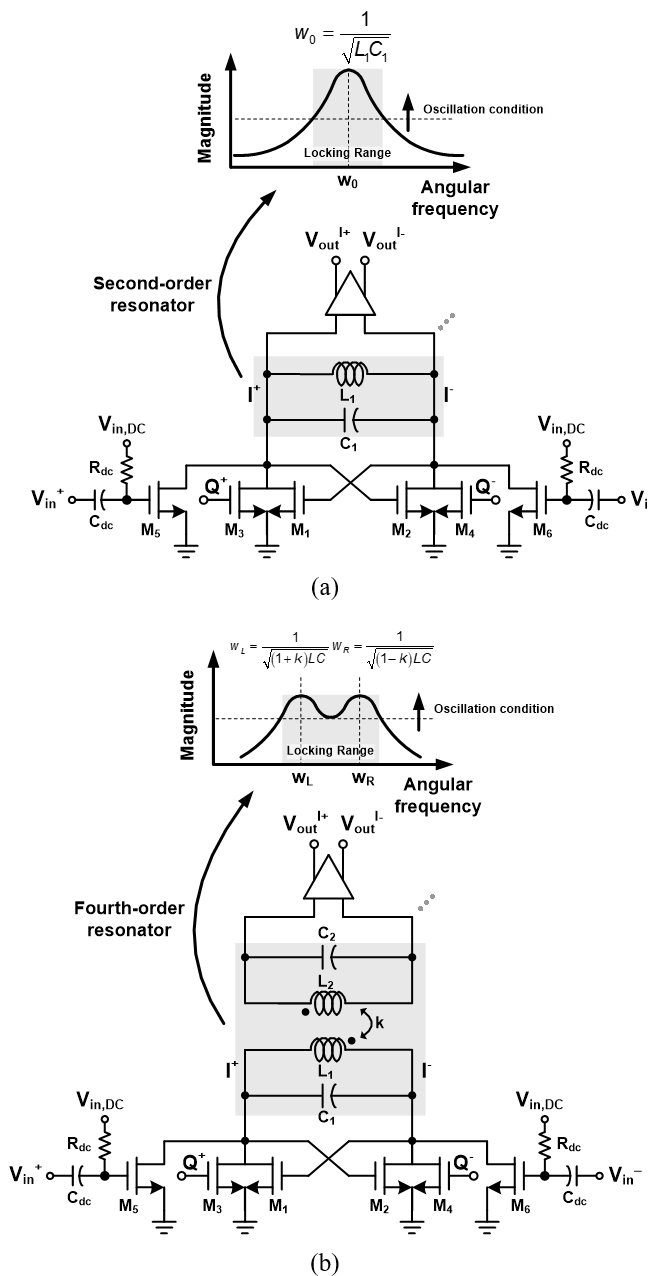Fig. 3 shows the load impedance magnitude of the IL-QVCO with a second-order resonator and fourth-order resonator, as well as a half core schematic. The second-order resonator consists of L1 and C1. Parasitic capacitors, including the drain–gate capacitors of M1–M6, are included in C1. This resonator has only one pole. The resonance angular frequency can be expressed as

##### (1)
$$w_{0}=\frac{1}{\sqrt{L_{1} C_{1}}}$$

The magnitude of the load impedance at w0 is the highest; however, the angular frequency range satisfied the oscillation condition is narrower than that of the fourth-order resonator (13,14). The fourth-order resonator comprises two poles. If the design parameter satisfies the following formula,

##### (2)
$$L_{1} \simeq L_{2} \simeq L, C_{1} \simeq C_{2} \simeq C$$

then the resonance angular frequency can be expressed as

##### (3)
$$w_{L}=\frac{1}{\sqrt{(1+k) L C}}, w_{R}=\frac{1}{\sqrt{(1-k) L C}}$$

where k is the coupling coefficient between L1 and L2. If the transformer is coupled strongly, the distance between the two poles increases, indicating that the locking range can be controlled by the coupling coefficient (15,16). Fig. 4 shows the simulation results of the impedance magnitude according to the coupling factor, k12. As the value of k12 increases, the distance between the two poles increases. Also, the self-resonant frequency is determined by the one with the higher impedance among the two poles. A wider operation frequency range can be obtained when a fourth-order resonator is used, rather than a second-order resonator, by adjusting the coupling coefficient. However, the locking range cannot be extended indefinitely because the IL-QVCO must satisfy the oscillation condition. The minimum oscillation condition follows the Barkhausen formula.

##### (4)
$$g_{m} \cdot\left|Z_{L}\right| \geq 1$$

where gm is the transconductance of the NMOS cross-coupled pair, and ZL is the magnitude of the load impedance of the IL-QVCO. As the distance between the two poles increases, a new minimum value is obtained. The IL-QVCO should be designed using this minimum value to satisfy the oscillation condition.

## III. MEASUREMENT RESULTS

This circuit was implemented by using a 65-nm complementary metal–oxide–semiconductor (CMOS) technology. Fig. 5 shows a photograph of the die. The chip size is 580 mm × 600 mm, and the entire die size, including all pads, is 860 × 770 mm. The power consumption of the core and output buffer are 42 and 11 mW, respectively. Fig. 6 shows the simulated and measured locking range of the IL-QVCO when Vdc is 0.7 V, with a supply voltage of 1 V. The simulated and measured locking ranges are 7.6–10.3 GHz and 7.08–10.56 GHz, respectively, when a 0 dBm input signal power is applied. The measurement result of the locking range is very similar to the simulation result. The self-resonance frequency difference between simulation and measurement is only 0.23 GHz.

Fig. 6. Simulated and measured locking range of the IL-QVCO: Vdc = 0.7 V and 1 V supply voltage.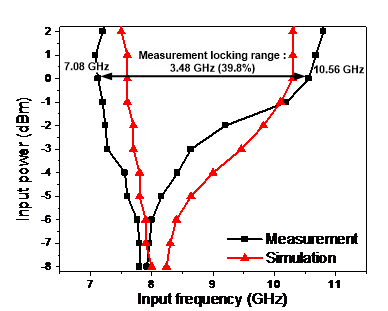Fig. 7 presents the Monte Carlo simulation results of the I/Q output signal when an 8 GHz injection signal is applied. The number of samples is 10000, mean phase difference is 0.643. The standard deviation is 4.87. Fig. 8 shows the measured I/Q output power and the amplitude imbalance according to the injection frequency. The maximum I/Q amplitude error is less than 2-dB.

Fig. 8. Measured I/Q output signal power and I/Q amplitude mismatch according to the injection frequency.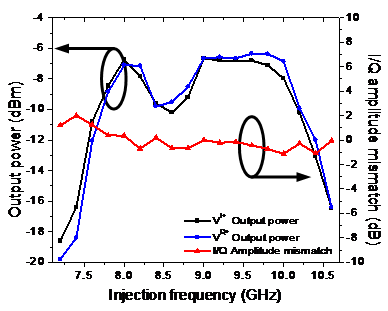Fig. 9. Spectrum when the minimum frequency injection signal is applied: 7.08 GHz.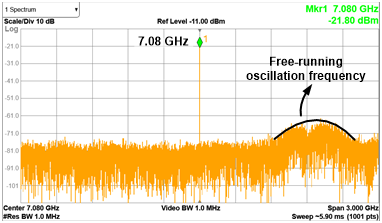Fig. 10. Spectrum when the maximum frequency injection signal is applied: 10.56 GHz.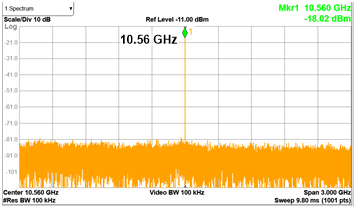Fig. 9 and 10 show the spectra when the minimum and maximum injection-locked frequencies are applied, respectively. The cables, connectors, and baluns used during the measurement produced an approximately 4-dB loss. Fig. 11 illustrates the phase noises for the cases when the 8 GHz injection-locked frequency is applied, and no input signal is applied. The self-oscillation frequency is 7.89 GHz, and the phase noise is −105.41 dBc/Hz.

Fig. 11. Measured I/Q output signal power and I/Q amplitude imbalance according to the injection frequency.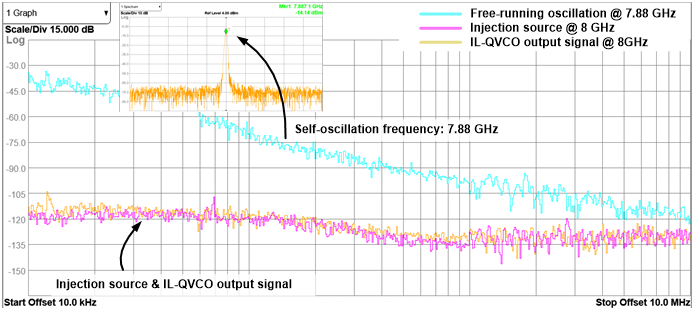Table 2. Performance comparison of other injection-locked circuits for generating quadrature signals

 (11) (17) (18) This work Technology 0.18 mm 0.18 mm 0.13 nm 65 nm Architecture SIC-QVCO VCO+ILFD ILFD+ILFM IL-QVCO Supply (V) 0.75 1.8 1.2 1.0 Output frequency (GHz) 5.11 – 5.87 2.2 – 3.2 19.6 – 20.4* 7.08 – 10.56 13.8% 37% 4% 39.8% PDC (mW) 16 – 26 16.5 48 42 Phase noise @ 100 kHz −99.8 dBc/Hz −96.8 dBc/Hz N/A −118.1 dBc/Hz @ 1 MHz −124.4 dBc/Hz −101.5 dBc/Hz −111 dBc/Hz −124.9 dBc/Hz Chip area (mm2) 0.81 N/A 1.8** 0.66

*: single side lock range > 350 MHz

**: full chip size

8 GHz injection-locked signal source has −125.38-dBc/Hz phase noise, and the IL-QVCO phase noise is −124.92 dBc/Hz. The phase noise values mentioned were at an offset frequency of 1 MHz. Table 2 shows the performance comparison of injection-locked circuits. Several blocks such as VCO, ILFD (injection-locked frequency divider), and ILFM (injection-locked frequency multiplier) are connected to generate quadrature signals (17,18). The phase noise in Table 2 is the value at each output frequency. The proposed IL-QVCO has the widest operation frequency range.

## IV. CONCLUSIONS

An IL-QVCO using a fourth-order resonator is implemented in a 65-nm CMOS technology. The fourth-order resonator is employed to generate wide frequency range of I/Q signals. The free-running frequency of the IL-QVCO is 7.89 GHz, and the input locking range is 7.08–10.56 GHz (39.8%) at an input power of 0 dBm. The I/Q phase difference is 0.6° in their mean value while the standard deviation reaches 4.87°. The phase noise is −124.92 dBc/Hz at an offset frequency of 1 MHz. The core power consumption was 42 mW from a supply voltage of 1 V. The die size was 0.86 mm × 0.77 mm.

### ACKNOWLEDGMENTS

This work was supported by Institute of Information & communications Technology Planning & Evaluation (IITP) grant funded by the Korea government(MSIT) (No. 2018-0-01663, Development of Communication-Sensing Converged B5G Millimeter Wave System) and Basic Science Research Program through the National Research Foundation of Korea (NRF) funded by the Ministry of Education (NRF-2020R1F1A1074076).

### REFERENCES

1
Wang Y., Jun 2019, A 39GHz 64-Element Phased-Array CMOS Transceiver with Built-in Calibration for Large-Array 5G NR, in IEEE RFIC, pp. 279-2822
Deng W., Aug, An Energy-Efficient 10-Gb/s CMOS Millimeter-Wave Transceiver With Direct-Modulation Digital Transmitter and I/Q Phase-Coupled Frequency Synthesizer, Solid-State Circuits, IEEE Journal of, Vol. 55, No. 8, pp. 2027-20423
Rimmelspacher J., Jun. 2020, Low Power Low Phase Noise 60 GHz Multichannel Transceiver in 28 nm CMOS for Radar Applications, in IEEE RFIC, pp. 19-224
Forsten H., Jul, Scalable 60 GHz FMCW Frequency-Division Multiplexing MIMO Radar, Microw. Theory Techn., IEEE Trans on., Vol. 68, No. 7, pp. 2845-28555
Lin Y., Apr, A Divide-by-Four Transformer-Coupled Regenerative Frequency Divider With Quadrature Outputs, Micro. Wireless Compon. Lett., IEEE, Vol. 24, No. 4, pp. 260-2626
R-Hosseinabadi N., Dehghani R., Khajehoddin S. A., Moez K., Jun 2020, A Wideband Frequency Divider With Programmable Odd/Even Division Factors and Quadrature/Symmetrical Outputs, Circuits and Systems I: IEEE Transactions on, Vol. 67, No. 6, pp. 1857-18667
Kaukovuori J., Nov 2008, Analysis and Design of Passive Polyphase Filters, Circuits and Systems II: IEEE Transactions on, Vol. 55, No. 10, pp. 3023-30378
Cetindogan B., Jan 2016, A 6 Bit Vector-Sum Phase Shifter With a Decoder Based Control Circuit for X-Band Phased-Arrays, Micro. Wireless Compon. Lett. IEEE, Vol. 26, No. 1, pp. 64-669
Jang S.-L., Huang S.-H., Liu C.-C., Juang M.-H., Apr 2009, CMOS Colpitts Quadrature VCO Using the Body Injection-Locked Coupling Technique, Micro. Wireless Compon. Lett. IEEE, Vol. 19, No. 4, pp. 230-23210
Jang S.-L., Huang S.-S., Lee C.-F., Juang M.-H., Oct 2008, CMOS Quadrature VCO Implemented With Two First-Harmonic Injection-Locked Oscillators, Micro. Wireless Compon. Lett. IEEE., Vol. 18, No. 10, pp. 695-69711
Lin S.-Y., Chiou H.-K., Mar 2019, A Modified High Phase Accuracy SIC-QVCO Using a Complementary-Injection Technique, Micro. Wireless Compon. Lett. IEEE., Vol. 29, No. 3, pp. 222-22412
Chamas I. R., Raman S., Mar 2009, Analysis and Design of a CMOS Phase-Tunable Injection-Coupled LC Quadrature VCO (PTIC-QVCO), Solid-State Circuits IEEE Journal of, Vol. 44, No. 3, pp. 784-79613
K.-I Oh , Ko G.-H., Kim J.-G., Baek D., Apr 2021, An 18.8–33.9-GHz, 2.26-mW Current-Reuse Injection-Locked Frequency Divider for Radar Sensor Applications, Sensors, Vol. 21, No. 255114
Oh K.-I., Ko G.-H., Kim G.-S., Kim J.-G., Baek D., May 2021, A 17.8–34.8 GHz (64.6%) Locking Range Current-Reuse Injection-Locked Frequency Multiplier with Dual Injection Technique, electronics, Vol. 10, No. 112215
Lin Y., Huang W., Lu C., Wang Y., Dec 2014, Wide-Locking-Range Multi-Phase-Outputs Regenerative Frequency Dividers Using Even-Harmonic Mixers and CML Loop Dividers, Microw. Theory Techn. IEEE Trans on., Vol. 62, No. 12, pp. 3065-307516
Zhang J., Aug 2018, Analysis and Design of Ultra-Wideband mm-Wave Injection-Locked Frequency Dividers Using Transformer-Based High-Order Resonators, Solid-State Circuits IEEE Journal of, Vol. 53, No. 8, pp. 2177-218917
Mangraviti G., Jul 2015, Design and Tuning of Coupled-LC mm-Wave Subharmonically Injection-Locked Oscillators, Microw. Theory Techn. IEEE Trans on., Vol. 63, No. 7, pp. 2301-231218
Jang S.-L., Chuang Y.-H., Lee S.-H., Lee C.-F., Feb 2007, An Integrated 5–2.5-GHz Direct-Injection Locked Quadrature LC VCO, Micro. Wireless Compon. Lett. IEEE, Vol. 17, No. 2, pp. 142-14419
Elbadry M., Sadhu B., Qiu J., Harjani R., Mar 2013, Dual-Channel Injection-Locked Quadrature LO Generation for a 4-GHz Instantaneous Bandwidth Receiver at 21-GHz Center Frequency, Microw. Theory Techn. IEEE Trans on., Vol. 61, No. 3, pp. 1186-1199## Author

Kwang-Il Oh was born in Gwang-myeong, Republic of Korea, in 1993.

He received his B.S. degree in physics and M.S. degree in electrical and electronics engineering from Chung-Ang University, Seoul, Re-public of Korea, in 2018 and 2020, respectively.

He is currently working toward his Ph.D. degree at the School of Electrical Engineering at Chung-Ang University.

His current research interests include CMOS RF transceivers for wireless communication and millimeter-wave radar.

Donghyun Baek (S’98–M’07–SM’13) received his B.S., M.S., and Ph.D. degrees in the department of electrical engineering, Korea Ad-vanced Institute of Science and Technology (KAIST), Daejeon, Korea, in 1996, 1998, and 2003, respectively.

From 2003 to 2007, he was with the System LSI Division of Samsung Electronics Company, Ki-heung, Korea, where he designed mobile broadcasting RF receivers, such as DVB-H, TDMB, and ISTB-T, and led the CMOS power amplifier project for handsets.

In 2007, he joined the School of Electrical Engineering, Chung-Ang University, Seoul, Korea, where he is currently an associate professor.

He is a life member of the IEEK and a senior member of the IEEE.

His research interests include analog, RF, and mixed-mode circuit designs for the mobile system on a chip(SOC), radar on a chip (ROC), and sensor on a chip (SOC).